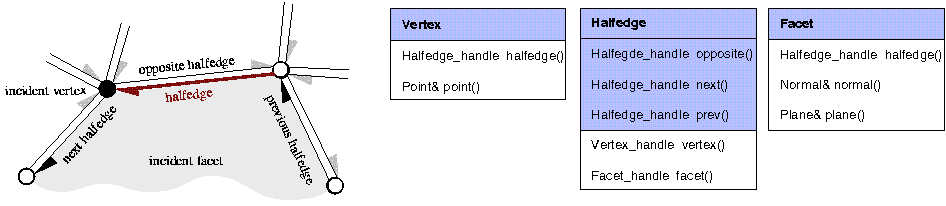CGAL 4.7 - 3D Polyhedral Surface
CGAL::Polyhedron_3< Traits >::Halfedge Class Reference

#include <CGAL/Polyhedron_3.h>

## Definition

A halfedge is an oriented edge between two vertices.

It is always paired with a halfedge pointing in the opposite direction. The Halfedge::opposite() member function returns this halfedge of opposite orientation. If a halfedge is incident to a facet the Halfedge::next() member function points to the successor halfedge around this facet. For border edges the Halfedge::next() member function points to the successor halfedge along the hole. For more than two border edges at a vertex, the next halfedge along a hole is not uniquely defined, but a consistent assignment of the next halfedge will be maintained in the data structure. An invariant is that successive assignments of the form h = h->next() cycle counterclockwise around the facet (or hole) and traverse all halfedges incident to this facet (or hole). A similar invariant is that successive assignments of the form h = h->next()->opposite() cycle clockwise around the vertex and traverse all halfedges incident to this vertex. Two circulators are provided for these circular orders.The three classes Vertex, Halfedge, and Facet of the polyhedral surface. Member functions with shaded background are mandatory. The others are optionally supported.

The incidences encoded in Halfedge::opposite() and Halfedge::next() are available for each instantiation of polyhedral surfaces. The other incidences are optionally available as indicated with type tags. The Halfedge::prev() member function points to the preceding halfedge around the same facet. It is always available, though it might perform a search around the facet using the Halfedge::next() member function to find the previous halfedge if the underlying halfedge data structure does not provide an efficient Halfedge::prev() member function for halfedges. Handles to the incident vertex and facet are optionally stored.

The circulators are assignable to the Halfedge_handle. The circulators are bidirectional if the halfedge provided to the polyhedron with the Items template argument provides a member function Halfedge::prev(), otherwise they are of the forward category.

CGAL::Polyhedron_3::Vertex
CGAL::Polyhedron_3::Facet
CGAL::Polyhedron_3<Traits>

Implementation

The member functions Halfedge::prev() and Halfedge::prev_on_vertex() work in constant time if Supports_halfedge_prev $$\equiv$$ CGAL::Tag_true. Otherwise both methods search for the previous halfedge around the incident facet.

## Types

typedef unspecified_type Vertex
type of incident vertices.

typedef unspecified_type Facet
type of incident facets.

typedef unspecified_type Vertex_handle
handle to vertex.

typedef unspecified_type Halfedge_handle
handle to halfedge.

typedef unspecified_type Facet_handle
handle to facet.

typedef unspecified_type Halfedge_around_vertex_circulator
circulator of halfedges around a vertex.

typedef unspecified_type Halfedge_around_facet_circulator
circulator of halfedges around a facet.

typedef unspecified_type Vertex_const_handle

typedef unspecified_type Halfedge_const_handle

typedef unspecified_type Facet_const_handle

typedef unspecified_type Halfedge_around_vertex_const_circulator

typedef unspecified_type Halfedge_around_facet_const_circulator

typedef unspecified_type Supports_halfedge_prev
$$\equiv$$ CGAL::Tag_true or CGAL::Tag_false.

typedef unspecified_type Supports_halfedge_vertex
$$\equiv$$ CGAL::Tag_true or CGAL::Tag_false.

typedef unspecified_type Supports_halfedge_face
$$\equiv$$ CGAL::Tag_true or CGAL::Tag_false.

## Creation

Halfedge ()
default constructor.

## Operations

Halfedge_handle opposite ()

Halfedge_const_handle opposite () const
the opposite halfedge.

Halfedge_handle next ()

Halfedge_const_handle next () const
the next halfedge around the facet.

Halfedge_handle prev ()

Halfedge_const_handle prev () const
the previous halfedge around the facet.

Halfedge_handle next_on_vertex ()

Halfedge_const_handle next_on_vertex () const
the next halfedge around the vertex (clockwise). More...

Halfedge_handle prev_on_vertex ()

Halfedge_const_handle prev_on_vertex () const
the previous halfedge around the vertex (counterclockwise). More...

bool is_border () const
is true if h is a border halfedge.

bool is_border_edge () const
is true if this or the opposite halfedge is a border halfedge.

Halfedge_around_vertex_circulator vertex_begin ()

Halfedge_around_vertex_const_circulator vertex_begin () const
circulator of halfedges around the vertex (clockwise), the vertex of the halfedges being *this.

Halfedge_around_facet_circulator facet_begin ()

Halfedge_around_facet_const_circulator facet_begin () const
circulator of halfedges around the facet (counterclockwise).

std::size_t vertex_degree () const
the degree of the incident vertex, i.e., number of edges emanating from this vertex.

bool is_bivalent () const
returns true if the incident vertex has exactly two incident edges.

bool is_trivalent () const
returns true if the incident vertex has exactly three incident edges.

std::size_t facet_degree () const
the degree of the incident facet, i.e., number of edges on the boundary of this facet.

bool is_triangle () const
returns true if the incident facet is a triangle.

returns true if the incident facet is a quadrilateral.

## Operations available if Supports_halfedge_vertex is CGAL::Tag_true

Vertex_handle vertex ()

Vertex_const_handle vertex () const
the incident vertex of the halfedge.

## Operations available if Supports_halfedge_facet is CGAL::Tag_true

Facet_handle facet ()

Facet_const_handle facet () const
the incident facet of the halfedge. More...

## Member Function Documentation

template<typename Traits >
 Facet_const_handle CGAL::Polyhedron_3< Traits >::Halfedge::facet ( ) const

the incident facet of the halfedge.

If the hafedge is a border halfedge the result is default construction of the handle.

template<typename Traits >
 Halfedge_const_handle CGAL::Polyhedron_3< Traits >::Halfedge::next_on_vertex ( ) const

the next halfedge around the vertex (clockwise).

Is equal to h.next()->opposite().

template<typename Traits >
 Halfedge_const_handle CGAL::Polyhedron_3< Traits >::Halfedge::prev_on_vertex ( ) const

the previous halfedge around the vertex (counterclockwise).

Is equal to h.opposite()->prev().